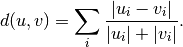# scipy.spatial.distance.canberra¶

scipy.spatial.distance.canberra(u, v)[source]

Computes the Canberra distance between two 1-D arrays.

The Canberra distance is defined asParameters : u : (N,) array_like Input array. v : (N,) array_like Input array. canberra : double The Canberra distance between vectors u and v.

Notes

When u[i] and v[i] are 0 for given i, then the fraction 0/0 = 0 is used in the calculation.

#### Previous topic

scipy.spatial.distance.braycurtis

#### Next topic

scipy.spatial.distance.chebyshev